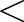Data Science Desktop Survival Guide by Graham WilliamsDesktop Survival Project Home Preface Data Science Introducing R R Constructs R Tasks R Strings R Read, Write, and Create Data Template Data Exploration Data Wrangling Data Visualisation Statistics ML Template ML Scenarios ML Activities ML Applications ML Algorithms Cluster Analysis Decision Trees Computer Vision Graph Data Privacy Literate Data Science Coding with Style Resources Bibliography Index

## Variables

20200501 We can store values, such as the resulting value from invoking a function (Section 3.5), into a variable. A variable is a name that we can use to refer to some location in the computer's memory. Into this location we store the data while our programs are running so that we can refer to that data by the specific variable name later on. We use the assignment operator base::- to do so:
 # Add two numbers and assign the result into a variable. v <- sum(1, 2) We can access the value stored in the variable (or actually stored in the computer's memory that the variable name refers to) simply by requesting through the R Console that R “run” the command v. We do so below with a line containing just `v`. In fact the R software actually runs the base::print() function to display the contents of the computer's memory that v refers to—this is a convenience that the R software provides for us.
# Print the value stored in a variable.

v
 ```##  3 ```
print(v)
 ```##  3 ```

By indexing the variable with `` we can ask for the first item referred to by v. Noting that the variable v is a vector with only a single item when we try to index a second item we get an `NA` (meaning not available or a missing value).

# Access a particular value from a vector of data.

v
 ```##  3 ```
v
 ```##  NA ```